# Geometry - Conic Sections

Hi all,

I am having an issue with the following problem. I just don't know how to approach it.

## Homework Statement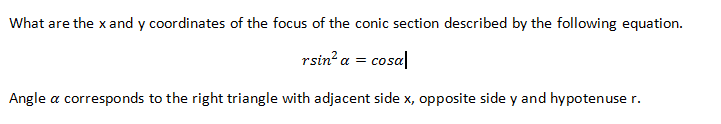## Homework Equations

Ax^2 + Bxy + Cy2 + Dx + Ey + F = 0

## The Attempt at a Solution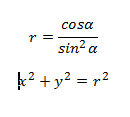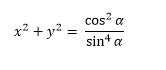I am confused on how to put this problem in terms of x & y and get numerical values for both x & y. Any guidance would be greatly appreciated :)

LCKurtz
Homework Helper
Gold Member
Hi all,

I am having an issue with the following problem. I just don't know how to approach it.

## Homework Statement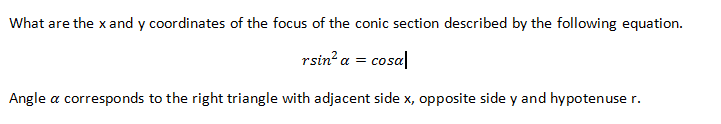## Homework Equations

Ax^2 + Bxy + Cy2 + Dx + Ey + F = 0

## The Attempt at a Solution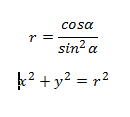I am confused on how to put this problem in terms of x & y and get numerical values for both x & y. Any guidance would be greatly appreciated :)

Try putting ##\sin\alpha = y/r## and ##\cos\alpha = x/r## in your equation.

Try putting ##\sin\alpha = y/r## and ##\cos\alpha = x/r## in your equation.
Great thank you. That was a big help and is starting to make sense now. Since it is a right triangle, your equations can be used.
$$x^{2}+y^{2} = \frac{(\frac{x}{r})}{(\frac{y}{r})^{2}}$$

$$\therefore x^{2}+y^{2} = \frac{(\frac{x}{r})}{(\frac{y}{r})^{2}}$$

$$\therefore x^{2}+y^{2} = (\frac{x}{r}) * \frac{y^{2}}{r^{2}}$$

$$\therefore x^{2}+y^{2} = (\frac{x}{r}) * \frac{r^{2}}{y^{2}}$$

$$\therefore x^{2}+y^{2} = ({x}) * \frac{r}{y^{2}}$$

I am still a little confused on how to get numerical values for x & y. This looks like it can be solved via the unit-circle if I set the right hand side of the equation to 1. Does this look right?

Thanks~

LCKurtz
Homework Helper
Gold Member
Great thank you. That was a big help and is starting to make sense now. Since it is a right triangle, your equations can be used.
$$x^{2}+y^{2} = \frac{(\frac{x}{r})}{(\frac{y}{r})^{2}}$$

$$\therefore x^{2}+y^{2} = \frac{(\frac{x}{r})}{(\frac{y}{r})^{2}}$$

$$\therefore x^{2}+y^{2} = (\frac{x}{r}) * \frac{y^{2}}{r^{2}}$$

That third step is wrong. To divide fractions you "invert and multiply". Also put in ##r^2## on the left side after you fix the right side.

LCKurtz
Homework Helper
Gold Member
Also, I missed that you had put ##x^2+y^2## on the left. That is ##r^2##. Your original equation just has ##r## and you should leave it there.

•1 person
That third step is wrong. To divide fractions you "invert and multiply". Also put in ##r^2## on the left side after you fix the right side.

Yes that was a typo. I actually do the correct method in step 4.

Also, I missed that you had put ##x^2+y^2## on the left. That is ##r^2##. Your original equation just has ##r## and you should leave it there.

I am getting the following:
$$r = \frac{x}{r} * \frac{r^{2}}{x^{2}}$$
$$cos \alpha = \frac{x}{r}/[tex] [tex]sin \alpha = \frac{y}{r}$$
$$r = \frac{\frac{x}{r}}{\frac{x^{2}}{r^{2}}}$$
$$r = \frac{x}{r} * \frac{r^{2}}{x^{2}}$$
$$r = \frac{x}{r} * \frac{r^{2}}{x^{2}}$$
$$r = \frac{xr}{y^{2}}$$
$$r = \frac{xr}{y^{2}}$$
$$1 = \frac{x}{y^{2}}$$

Stuck again...now a little confused. Do I need to put this in the form $$x^{2} + y^{2} = r^{2}$$

LCKurtz
Homework Helper
Gold Member
I am getting the following:

$$1 = \frac{x}{y^{2}}$$

Stuck again...now a little confused. Do I need to put this in the form $$x^{2} + y^{2} = r^{2}$$

You want an x-y equation. What's wrong with ##x=y^2##? Now use what you know about xy conics to locate its focus.

Great. I think I got it. Since this equation is in the form of:
$$x=y^{2}$$
it is a parabola with the general equation of $$x = a(y-k)^{2} + h$$. a = 1 and k = 0 in this case. The focus point for a parabola in this form is at $$(h+p, k)$$ And $$p = \frac{1}{4a}$$. Therefore, since a = 1, $$p=\frac{1}{4}$$.

The focus point is therefore $$(\frac{1}{4}, 0)$$

Thanks LCKurtz!